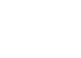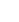# Statistics Operations (PEMDAS/BEDMAS): Easy Steps

Probability and Statistics > Statistics Operations (PEMDAS/BEDMAS)In order to succeed in statistics, you’re going to have to be familiar with Order of Operations. The “operations” are: addition, multiplication, division, subtraction, exponents, and grouping. They can be performed in multiple ways, and if you don’t follow the “order”, you’re going to get the problem wrong. For example:

4 + 2 × 3

can equal 18 or 10, depending on whether you do the multiplication first or last:

4 + 2 × 3
6 × 3
18 (Wrong!)

4 + 2 × 3
4 + 6
10 (Right!)

This article shows you how to get it right every time with an acronym: PEMDAS.

## Statistics Operations: What Does PEMDAS Mean?

The acronym PEMDAS actually stands for Parentheses, Exponents, Multiplication, Division, Addition, and Subtraction, but you may easily remember it as “Please Excuse My Dear Aunt Sally”.

## What is BEDMAS?

BEDMAS is identical for all practical purposes; the “B” is for “brackets or parentheses”. As you’ll see brackets[] or parentheses () in many equations, BEDMAS makes it arguably clearer what order to perform operations involving either of those two. In BEDMAS, division comes before multiplication (in PEMDAS, multiplication comes first). It doesn’t make any difference, as those two operations can be performed in any order.

## Statistics Operations: Example

Let’s say we have a question that asks you to solve the following equation:

1.96 + z whereYou are given:= 5,
μ= 3 × 7,
s = 52,
n = 100.

Step 1: Insert the numbers into the equation:
1.96 + z = 1.96 + ((5 – (3 × 7)) / (52 / √100)*)
or
1.96 + z = 1.96 + ((5 – (3 × 7)) / 52 / 10))**

Step 2: Perform the first operation: Parentheses. Work from the inside out if there are multiple parentheses. The inside parentheses is 3 × 7 = 21, so:
1.96 + z = 1.96 + ((5 – 21) / (52 / 10))
and there’s only one operation left inside that first set of parentheses, so let’s go ahead and do it:
1.96 + z = 1.96 + ((-16) / (52 / 10))
Next, we tackle the second set of parentheses.

Step 3: Perform the second operation: Exponents.
52 = 25, so:
1.96 + z = 1.96 + (-16 / (25 / 10))

Step 4: Perform the third operation. There’s no Multiplication in this equation, so let’s do the next one: Division. Notice there are two division signs. You may be wondering which one you should do first.  Remember that we have to evaluate everything inside parentheses first, so we can’t do the first division sign on the left until we evaluate (25 / 10), so let’s do that:
25 / 10 = 2.5
1.96 + z = 1.96 + (-16/(2.5))

Now we can do the second division: -16 / 2.5 = -6.4 (thanks for the correction, Dean!)

Step 5: 1.96 + (-6.4) = -4.44

Step 6: Do the Subtraction. In this case there is nothing more to do, so we’re already done!

This should hopefully jog your memory–PEMDAS and Order of Operations is covered in basic math classes and you should be thoroughly familiar with the concept in order to succeed with statistics.

Notes:

* Notice that I placed parentheses around the top and bottom parts of the equation to separate them. If I hadn’t, the equation would look like this:
z = 5 – 3 × 7 / 52 / √100
which could easily be interpreted (incorrectly) as this:
z = (5 – 3 × 7 / 52) / √100
so use parentheses liberally, as a reminder to yourself which parts of the equation go together.

** √100 = 10. You don’t need PEMDAS to evaluate simple square roots like this one.

## References

Gonick, L. & Smith, W. The Cartoon Guide to Statistics. HarperPerennial.
Zegarelli, M. (2012). Basic Math and Pre-Algebra For Dummies.

CITE THIS AS:
Stephanie Glen. "Statistics Operations (PEMDAS/BEDMAS): Easy Steps" From StatisticsHowTo.com: Elementary Statistics for the rest of us! https://www.statisticshowto.com/statistics-basics/statistics-operations-pemdas-bedmas/
---------------------------------------------------------------------------Need help with a homework or test question? With Chegg Study, you can get step-by-step solutions to your questions from an expert in the field. Your first 30 minutes with a Chegg tutor is free!